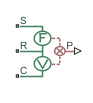# Translational Power Sensor

Average or instantaneous translational power sensor

•Libraries:
Simscape / Driveline / Sensors

## Description

The Translational Power Sensor block represents a sensor that measures the translational power change due to one or more driveline components connected between ports R and C. The power output can be an instantaneous or average value. Two averaging options are available: period and vibration averaging.

The instantaneous power is the product of the current velocity and force. Use the instantaneous power to analyze peak power transmission and identify heavily loaded components.

The time-averaged power is the integral of the instantaneous power over one sample period, divided by that period. For simulation times smaller than the sample period, the average power is zero. The power is constant for each sample period.

The vibration-averaged power is the integral of the power over one vibration period, divided by that period. The vibration period is the time between two consecutive crossings from negative to positive velocity values. Use this averaging method only for oscillations with velocity passing through zero. If the vibration has a variable frequency, the averaging period can change. For simulation times smaller than the vibration period, the average power is zero. The power output is constant for each vibration period.

## Ports

### Input

expand all

Physical signal input port associated with the translational power, in W.

### Conserving

expand all

Mechanical translational conserving port associated with the driving source.

Mechanical translational conserving port associated with the rod or shaft.

Mechanical translational conserving port associated with the case.

## Parameters

expand all

Whether to measure instantaneous power, average power over a specified period, or average power over a vibration.

• `Instantaneous power` — Output the power measured for the current time step.

• `Average power over a specified period` — Output the power averaged over a fixed time period.

• `Average power over a vibration` — Output the power averaged over one vibration period.

Time period over which to average the power. Typical values include the cycle length of a reciprocating device or the actuation force.

Maximum vibration frequency over which to average the power. Very high values tend to reduce simulation speed. Very low values tend to result in lower power measurements.

## Version History

Introduced in R2013a